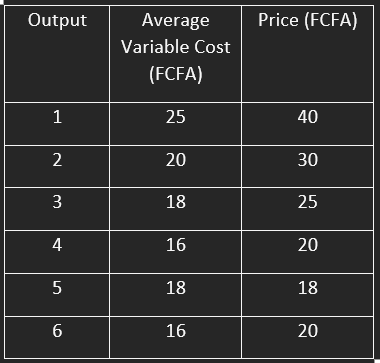# A Level Economics Quiz 2019 Part 2

#### Quiz Description

Economics includes the study of labor, land and investments, of money, income and production, and of taxes and government expenditures.

Just from its definition we can automatically say that it deals with a lot of mathematics and reasoning. Bringing these calculations to an exam, it becomes a huge problem for most advanced students. In a way to help out, we have designed a quiz of 15 questions. This quiz aims principally at testing students who are preparing for the upcoming session of the CGCE Advanced level.

The questions present are questions from the June session of 2019 Advanced Level Economics. We can bear in mind that the best way to prepare for an exam is to solve its past questions. With a focus, a student will be able to go through this quiz and ace all the questions.Upon completion of this quiz, you can move on to the next session. The next quiz is the continuation of the year 2019 and it also contains 15 questions.

In pure monopoly, price is:

Correct
• Greater than marginal revenue
• Less than marginal revenue
• Greater than average revenue
• Less than average revenue

In a perfectly competitive market, what short run decision will you advise this firm to take at output 6?Correct
• Increase output price
• Reduce output price
• Continue production
• Stop production

The main feature of firms in the monopolistic market is:

Correct
• Collusion
• Interdependence
• Product differentiation
• Price discrimination

If the marginal revenue is equal to zero in the monopoly market, the value of price elasticity of demand will be:

Correct
• One
• Zero
• Less than one
• Greater than one

A national industry aiming at financial autonomy will charge the price where:

Correct
• MC=MR
• AR=MC
• AR=AC
• AC=MC

If price is less than MC, a firm in perfect competition could increase profit by:

Correct
• Increasing output
• Decreasing output
• Decreasing inputs
• Increasing the inputs

The minimum payment required to maintain a factor of production in the same line of production is called:

Correct
• Quasi rent
• Transfer earning
• Transfer payment
• Economic rent

Wages differ within the same occupation because

Correct
• Of differences in profitability
• Experience is considered for payment
• Of differences in bargaining strength of trade unions
• Of occupational immobility

This question is based on table 3 which shows the rate of interest and idle balances in a given economy in a certain year. The supply of money is 1,000

What amount of active balance will give rise to an 8% equilibrium rate of interest?

Correct
• 600
• 300
• 400
• 200

Normal profit is:

Correct
• The supply of entrepreneurship
• Profit earned where MR=MC
• The positive difference between TR and TC
• Profit earned where TR is greater than TC

Double counting can be avoided by:

Correct
• Including the value of second hand goods
• Including the value of second hand goods
• Considering only the value added at each stage of production

The problem of using other countries’ resources when measuring national income can be solved by:

Correct
• Reducing imports and adding exports
• Using net property income from abroad
• Adding subsidies and subtracting taxes
• Adding taxes and subtracting subsidies

This question is based on the statistics below:

Gross national product at market prices =2,000 million FCFA

Net property income from abroad =-20 million FCFA

Indirect taxes =160 million FCFA

Subsidies =100 million FCFA

Capital stock =8,000 million FCFA

Depreciation = 10% of capital stock.

The value of national income is:

Correct
• 1,940 million FCFA
• 1,940 million FCFA
• 1,180 million FCFA
• 1,140 million FCFA

The real difference between real national income and money national income is that:

Correct
• Money national income considers goods and services unlike real national income
• Money national income is measured at constant process unlike real national income
• Real national income changes over time unlike money national income
• Real national income is measured at constant prices unlike money national income

The paradox of thrift assumes that:

Correct
• Money borrowed is reinvested
• Money laundering exists
• There is unemployment
• The economy is expanding rapidly